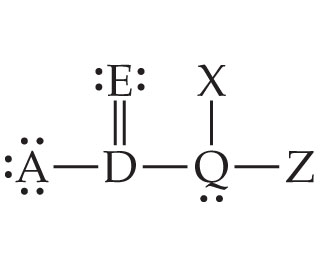# Problem: In the Lewis structure shown here, A, D, E, Q, X, and Z represent elements in the first two rows of the periodic table (H - Ne).Identify Q so that its formal charge is zero.

###### FREE Expert Solution

Formal Charge is the charge assigned to an atom in a molecule, assuming that electrons in all chemical bonds are shared equally between atoms, regardless of relative electronegativity.

The steps that have to be taken to solve this problem are:

1. Use the formal charge equation to find the group number.
2. Note that the formal charge around Q is zero.
88% (398 ratings)###### Problem Details
In the Lewis structure shown here, A, D, E, Q, X, and Z represent elements in the first two rows of the periodic table (H - Ne).Identify Q so that its formal charge is zero.

Frequently Asked Questions

What scientific concept do you need to know in order to solve this problem?

Our tutors have indicated that to solve this problem you will need to apply the Formal Charge concept. You can view video lessons to learn Formal Charge. Or if you need more Formal Charge practice, you can also practice Formal Charge practice problems.